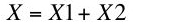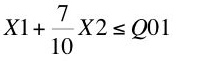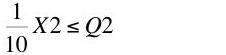## Sunday, February 04, 2007

### Example With Heterogeneous Labor (Part 1 of 3)

1.0 Introduction
I thought I would go through another example in which firms want to hire more workers (per unit output) at a higher wage. Since the last time I presented such an example, I've learned more about how to present mathematics in a blog post.

This example was originally developed by Metcalfe and Steedman. I've renamed the inputs so as to present it as a case of non-competing types of heterogeneous labor. I don't think reswitching need occur for the behavior illustrated in Figure 5 in the third part to occur.

2.0 Data On Technology
Consider a very simple economy that produces a single consumption good, corn, from inputs of two categories of labor, steel, and (seed) corn. All production processes in this example require a year to complete. Two production processes are known for producing steel. These processes require the inputs shown in Table 1 to be available at the start of the year for each ton steel produced and available at the end of the year. Two processes, as shown in Table 2, are also known for producing corn.
 Process A Process B Category 1 Labor (1/100) Person-Year (33/50) Person-Year Category 2 Labor 1 Person-Year (6/5) Person-Year Steel 0 Ton (3/10) Ton Corn (71/100) Bushel (1/50) Bushel

 Process C Process D Category 1 Labor 1 Person-Year (7/10) Person-Year Category 2 Labor 1 Person-Year (4/5) Person-Year Steel (1/10) Ton (1/5) Ton Corn 0 Bushel (1/10) Bushel
A technique consists of a process for producing the consumption good, corn, and a process for producing each non-consumption reproducible good used as an input in the process for producing the consumption good. In other words, a technique is a combination of one steel-producing process and one corn-producing process. The number of techniques is the product of the number of corn-producing processes and the number of steel-producing process. Thus, four techniques exist in this example. They are defined in Table 3.
 Technique Processes Alpha A, D Beta A, C Gamma B, C Delta B, D

Appendix A
The production functions that describe the technology in this example exhibit common neoclassical features: Constant Returns to Scale and non-increasing marginal returns to each factor. For purposes of constructing the production function for corn, the quantities of both categories of labor, steel, and corn available as input to corn production are taken as given. Let Q01, Q02, Q1, and Q2 be these quantities of category one labor, category two labor, steel, and corn, respectively. All quantities are measured in physical units (person-years, tons, bushels). The following Linear Program expresses the problem of maximizing the output of corn from these inputs: choose X1 and X2, the quantities of corn produced by processes C and D, respectively, to maximize:(A-1)
such that(A-2)(A-3)(A-4)(A-5)(A-6)
The production function for corn, f( Q01, Q02, Q1, Q2 ) is the value of the objective function, X* for the solution of the above Linear Program, expressed as a function of the physical inputs into corn production. These parameters define the right-hand-side of the linear constraints in Displays A-2 through A-5. The left-hand-side of these constraints is defined by the parameters in Table 2.

One standard method of visualizing production functions is with isoquants. An isoquant for this production function is a four dimensional surface, which I find difficult to draw. Accordingly, suppose the inputs of category 1 labor and corn are not binding for the level of output for which isoquants are drawn. Then Figure A-1 shows the isoquants for the corn production function in the remaining two dimensions. The dashed rays from the origin correspond to the two processes available for producing corn. If only one process for producing corn was known (a Leontief production function or "fixed coefficients" of production), an isoquant would consist of two rays, one extending horizontally right from the dashed line and the other extending vertically upward from the dashed line for that process. For the two known processes, an isoquant also contains the line segment shown sloping downward to the right. This line segment corresponds to a linear combination of the processes C and D, in which coefficients of production continuously vary.Figure A-1: Isoquants For Corn Production Function
One can also consider the (physical) marginal product of category 2 labor as the slope of the function shown in Figure A-2. In this case, no inputs except category 2 labor are binding for the lowest quantities of category 2 labor input. Since process D produces more corn than process C per person-year of category 2 labor, process D provides the steepest part of this projection of the production function for corn. When the steel input becomes binding, the marginal product of category 2 labor declines to the slope of the line segment corresponding to linear combinations of the two processes. Finally, the other inputs constrain a corn-producing firm to only adopt process C for a fixed level of output, and the marginal product of category 2 labor is zero. For this model of technology the marginal product of each input is a decreasing step function.Figure A-2: Outputs As Category 2 Labor Varies
A textbook presentation of production functions to students assumed to know the calculus often presents smooth isoquants and production functions that are at least twice continuously differentiable. A discrete model of technology can approximate smooth functions arbitrarily closely, given enough processes. When more processes are available, more line segments representing linear combinations of processes arise. Smooth production functions correspond to the limit, in which there an uncountably infinite number of processes available. As I understand it, no point in the limit case, however, corresponds to a linear combination of processes. "Perverse" Sraffa effects can arise in both a discrete and a smooth technology. But I like Linear Programming, and will stick to a discrete model of technology.

#### 1 comment:

Anonymous said...

Whereas in the Sraffian theory a single technology is always most efficient (except in the switching point), the optimization problems with scarce resources may yield solutions with a mixed technology. Apparently the maximization of the objective function, for instance with linear programming, does not guarantee the realization of the largest profit rate. The maximization may result in the use of inefficient technology. This is true even when the function is the value of the net product itself. In addition the mix reacts tot scarcity by means of substitution. I wonder what this means or implies.